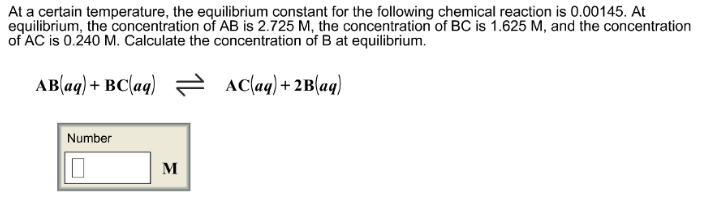Chemistry Practice Problems Equilibrium Expressions Practice Problems Solution: At a certain temperature, the equilibrium constant...

🤓 Based on our data, we think this question is relevant for Professor Hurst's class at GS.

# Solution: At a certain temperature, the equilibrium constant for the following chemical reaction is 0.00145. At equilibrium, the concentration of AB is 2.725 M, the concentration of BC is 1.625 M, and the concentration of AC is 0.240 M. Calculate the concentration of B at equilibrium. AB (aq) + BC (aq) ⇌ AC (aq) + 2B (aq)

###### Problem

At a certain temperature, the equilibrium constant for the following chemical reaction is 0.00145. At equilibrium, the concentration of AB is 2.725 M, the concentration of BC is 1.625 M, and the concentration of AC is 0.240 M. Calculate the concentration of B at equilibrium.

AB (aq) + BC (aq) ⇌ AC (aq) + 2B (aq)View Complete Written Solution

Equilibrium Expressions

Equilibrium Expressions

#### Q. What is the numerator for the equilibrium expression, Kc, for the following chemical equilibrium? Cu2+ (aq) + 4NH3 (aq) = Cu(NH3)42+ (aq) a. [Cu(NH3)4...

Solved • Wed Aug 01 2018 13:07:19 GMT-0400 (EDT)

Equilibrium Expressions

#### Q. Nitric oxide and bromine were allowed to react in a sealed container. When equilibrium was reached PNO = 0.526 atm = 1.59 atm, and PNOBr = 12.2 atm. C...

Solved • Wed Aug 01 2018 12:59:55 GMT-0400 (EDT)

Equilibrium Expressions

#### Q. The equilibrium constant is 9.36 x 10-17 at 25 °C. The reaction is endothermic with an enthalpy of 131 kJ/mol. What is the equilibrium constant at 700...

Solved • Wed Aug 01 2018 12:55:55 GMT-0400 (EDT)

Equilibrium Expressions

#### Q. The equilibrium between hemoglobin and oxyhemoglobin in the blood can be represented by the following reaction. Write the form of the equilibrium cons...

Solved • Mon Jul 23 2018 15:56:07 GMT-0400 (EDT)